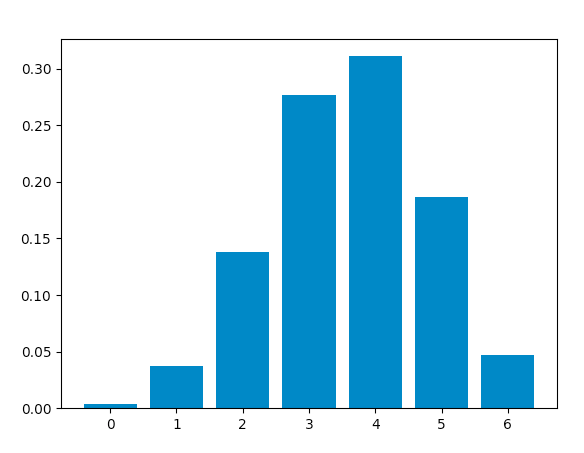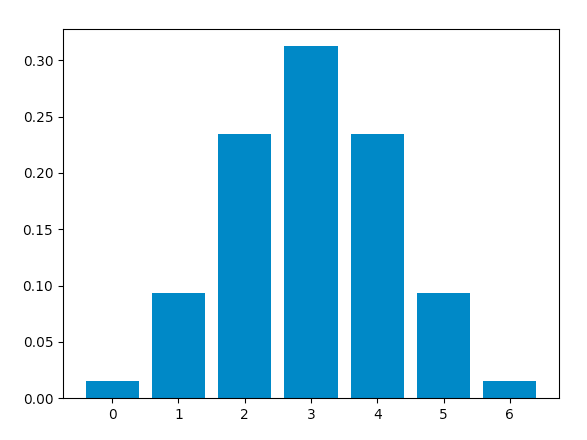# Python – Binomial Distribution

Binomial distribution is a probability distribution that summarises the likelihood that a variable will take one of two independent values under a given set of parameters. The distribution is obtained by performing a number of Bernoulli trials.

A Bernoulli trial is assumed to meet each of these criteria :

• There must be only 2 possible outcomes.
• Each outcome has a fixed probability of occurring. A success has the probability of p, and a failure has the probability of 1 – p.
• Each trial is completely independent of all others.

The binomial random variable represents the number of successes(r) in n successive independent trials of a Bernoulli experiment.

Probability of achieving r success and n-r failure is :The number of ways we can achieve r successes is :Hence, the probability mass function(pmf), which is the total probability of achieving r success and n-r failure is :An example illustrating the distribution :

Consider a random experiment of tossing a biased coin 6 times where the probability of getting a head is 0.6. If ‘getting a head’ is considered as ‘success’ then, the binomial distribution table will contain the probability of r successes for each possible value of r.

This distribution has a mean equal to np and a variance of np(1-p)

Using Python to obtain the distribution :
Now, we will use Python to analyse the distribution(using SciPy) and plot the graph(using Matplotlib).
Modules required :

• SciPy:
SciPy is an Open Source Python library, used in mathematics, engineering, scientific and technical computing.

Installation :

pip install scipy

• Matplotlib:
Matplotlib is a comprehensive Python library for plotting static and interactive graphs and visualisations.

Installation :

pip install matplotlib


The scipy.stats module contains various functions for statistical calculations and tests. The stats() function of the scipy.stats.binom module can be used to calculate a binomial distribution using the values of n and p.

Syntax : scipy.stats.binom.stats(n, p)

It returns a tuple containing the mean and variance of the distribution in that order.

scipy.stats.binom.pmf() function is used to obtain the probability mass function for a certain value of r, n and p. We can obtain the distribution by passing all possible values of r(0 to n).

Syntax : scipy.stats.binom.pmf(r, n, p)

Calculating distribution table :

Approach :

• Define n and p.
• Define a list of values of r from 0 to n.
• Get mean and variance.
• For each r, calculate the pmf and store in a list.

Code :

 from scipy.stats import binom  # setting the values  # of n and p  n = 6 p = 0.6 # defining the list of r values  r_values = list(range(n + 1))  # obtaining the mean and variance   mean, var = binom.stats(n, p)  # list of pmf values  dist = [binom.pmf(r, n, p) for r in r_values ]  # printing the table  print("r\tp(r)")  for i in range(n + 1):      print(str(r_values[i]) + "\t" + str(dist[i]))  # printing mean and variance  print("mean = "+str(mean))  print("variance = "+str(var))

Output :

r    p(r)
0    0.004096000000000002
1    0.03686400000000005
2    0.13824000000000003
3    0.2764800000000001
4    0.31104
5    0.18662400000000007
6    0.04665599999999999
mean = 3.5999999999999996
variance = 1.44


Code: Plotting the graph using matplotlib.pyplot.bar() function to plot vertical bars.

 from scipy.stats import binom  import matplotlib.pyplot as plt  # setting the values  # of n and p  n = 6 p = 0.6 # defining list of r values  r_values = list(range(n + 1))  # list of pmf values  dist = [binom.pmf(r, n, p) for r in r_values ]  # plotting the graph   plt.bar(r_values, dist)  plt.show()

Output :When success and failure are equally likely, the binomial distribution is a normal distribution. Hence, changing the value of p to 0.5, we obtain this graph, which is identical to a normal distribution plot :Attention geek! Strengthen your foundations with the Python Programming Foundation Course and learn the basics.

To begin with, your interview preparations Enhance your Data Structures concepts with the Python DS Course.

My Personal Notes arrow_drop_upCheck out this Author's contributed articles.

If you like GeeksforGeeks and would like to contribute, you can also write an article using contribute.geeksforgeeks.org or mail your article to contribute@geeksforgeeks.org. See your article appearing on the GeeksforGeeks main page and help other Geeks.

Please Improve this article if you find anything incorrect by clicking on the "Improve Article" button below.

Article Tags :
Practice Tags :

1

Please write to us at contribute@geeksforgeeks.org to report any issue with the above content.